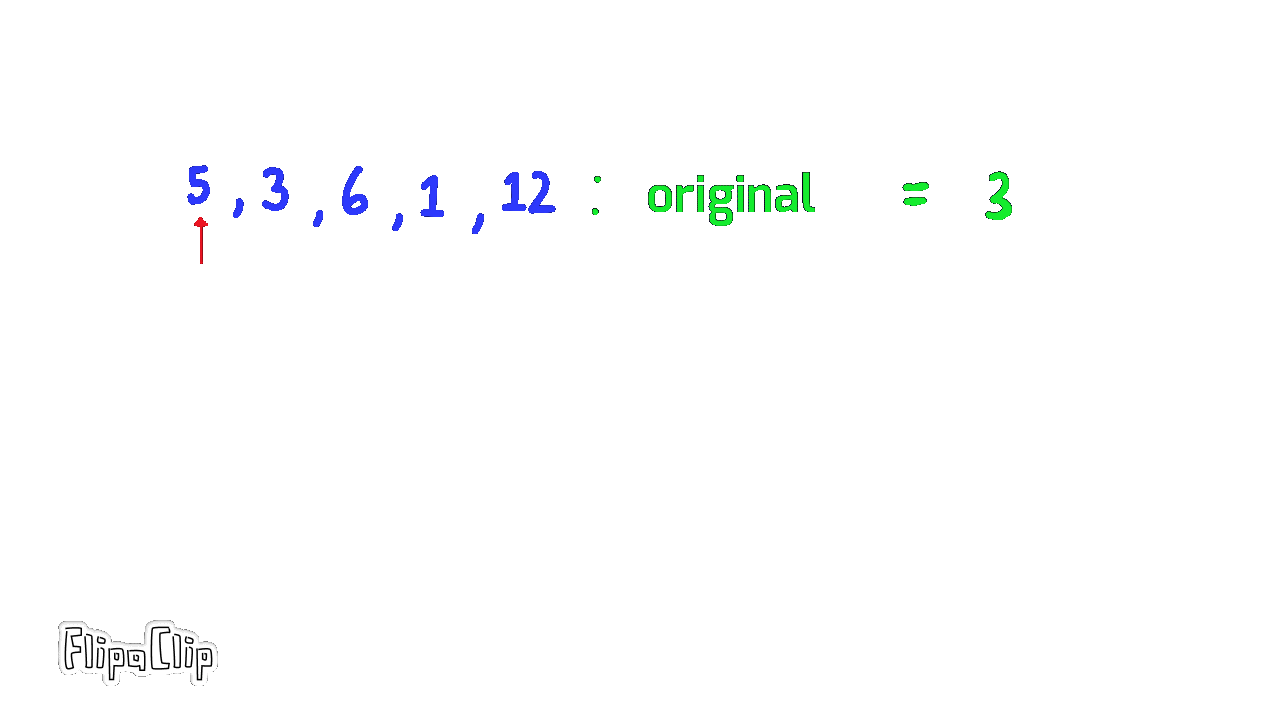2154

Intiution

• We can iterate throught the whole array, if we find an element the same as original, we multiply original by 2 and reiterate through the the array with the new original .``````func findFinalValue(nums []int, original int) int {
for i := 0 ; i < len(nums) ; i++{
if nums[i] == original {
original *= 2
i = -1
}
}
return original
}
``````